# RD Sharma Solutions for Class 8 Maths Chapter 2 - Powers Exercise 2.2

In Exercise 2.2 of RD Sharma Class 8 Maths Chapter 2 Powers, we shall discuss problems based on the decimal number system and laws of integral exponents. RD Sharma Class 8 Solutions for Maths are solved by our experts in order to help students in learning the concepts effortlessly from the Maths textbook. Students can easily download the pdf of Exercise 2.2 Solutions from the links provided below.

## Download PDF of RD Sharma Solutions for Class 8 Maths Exercise 2.2 Chapter 2 Powers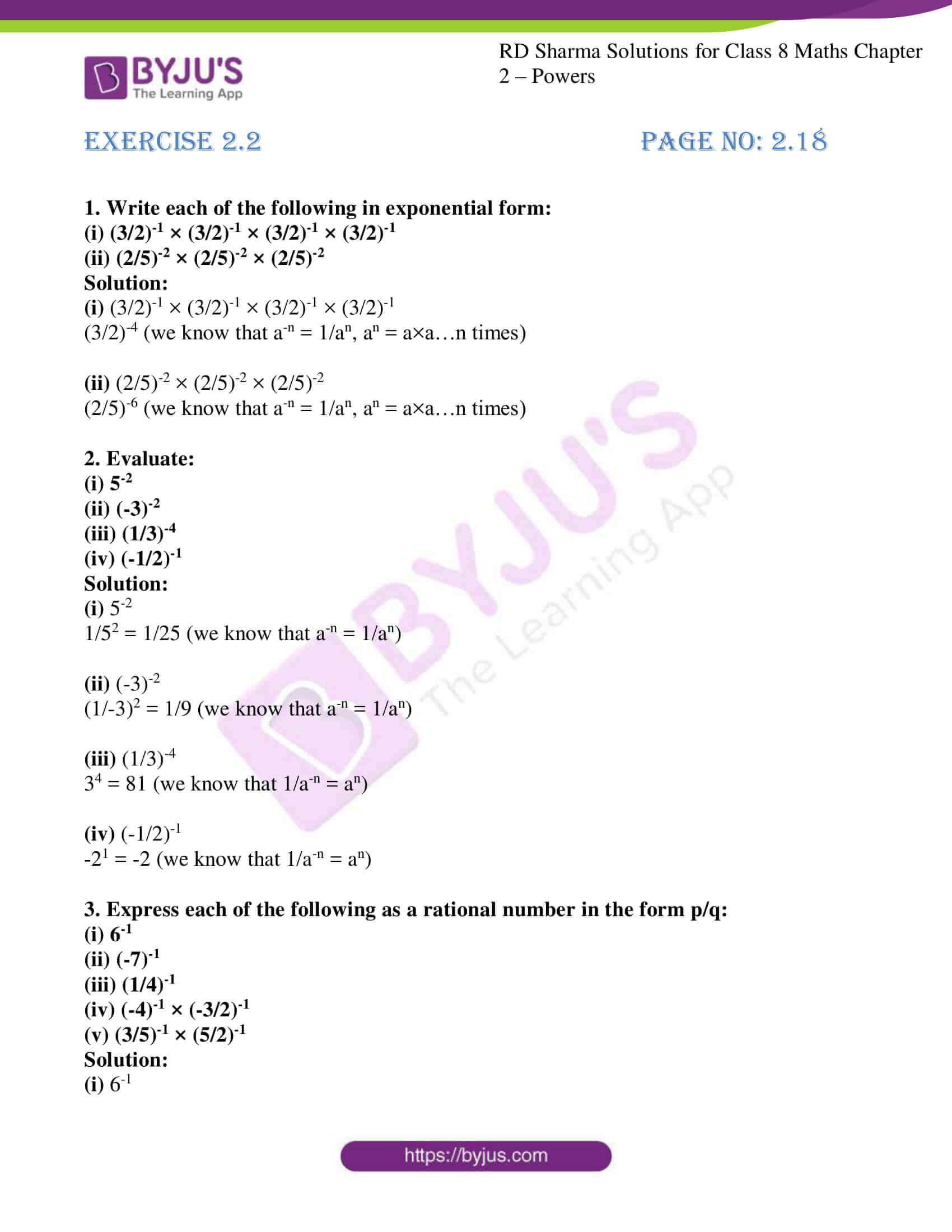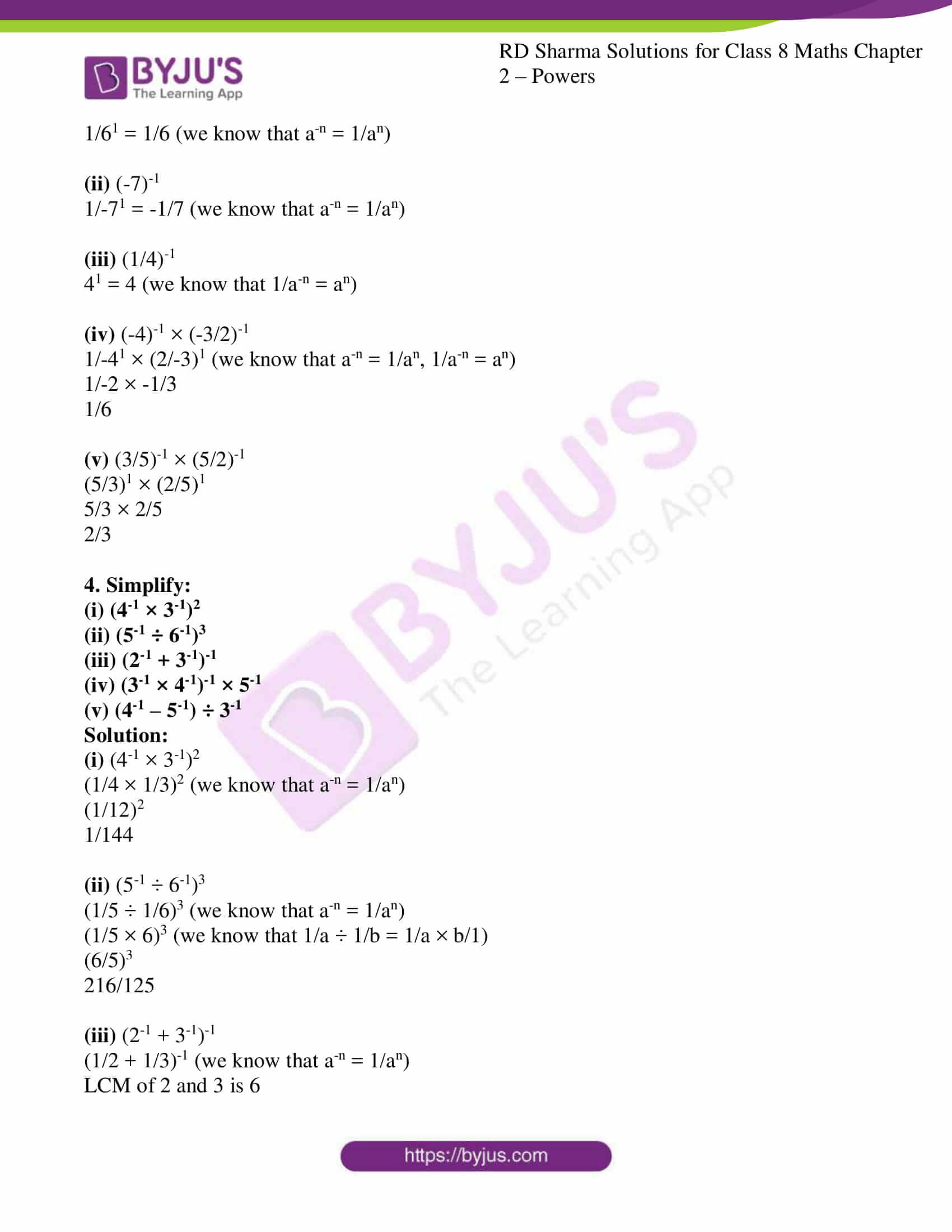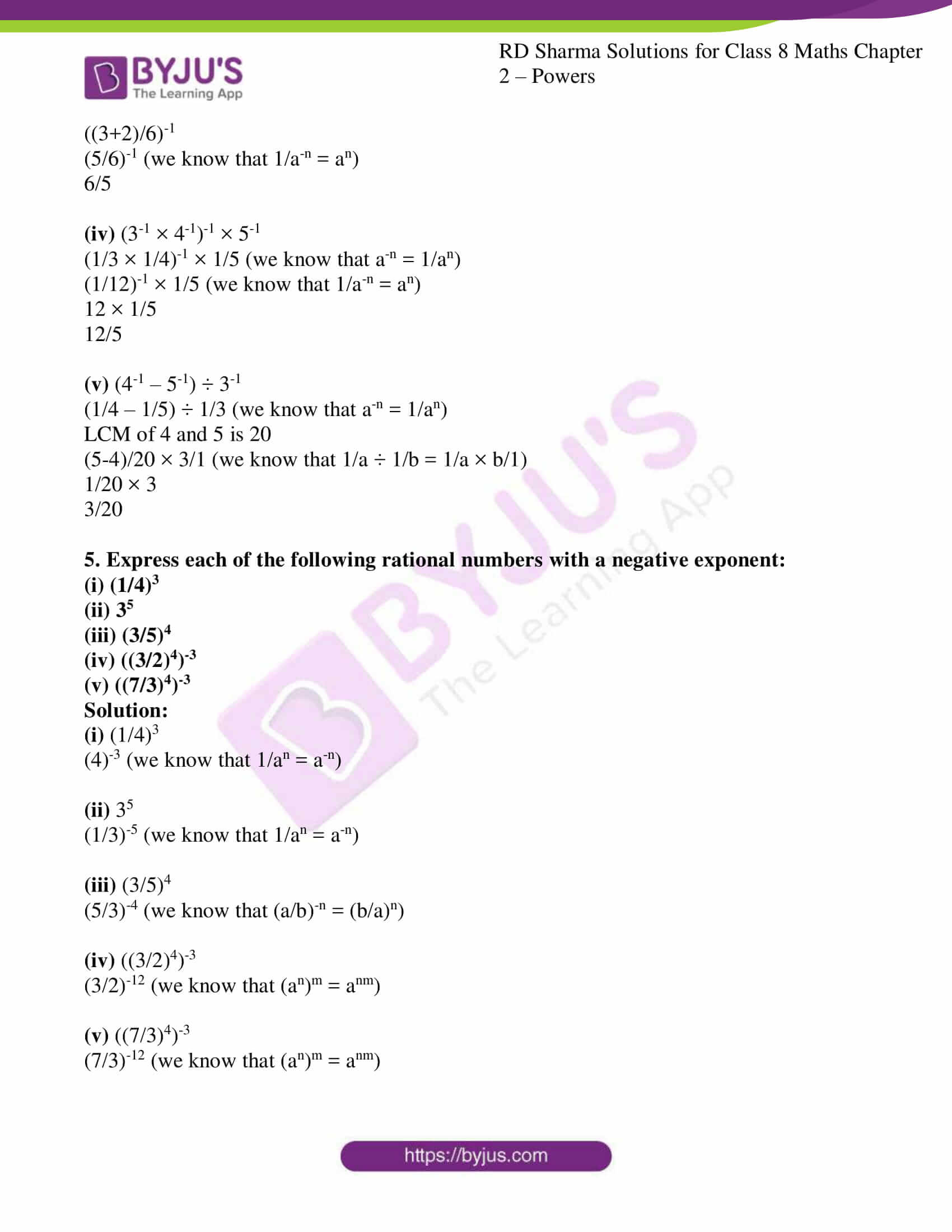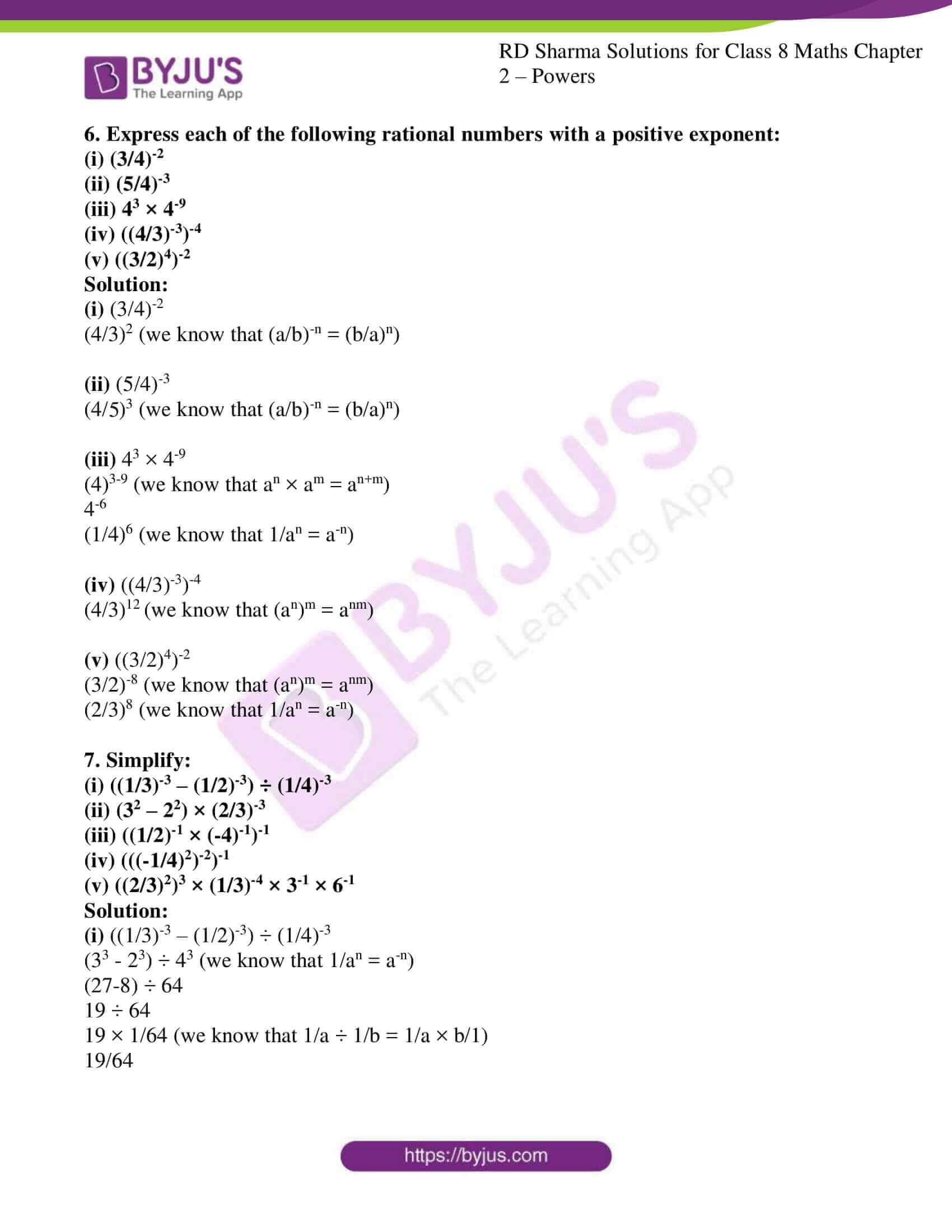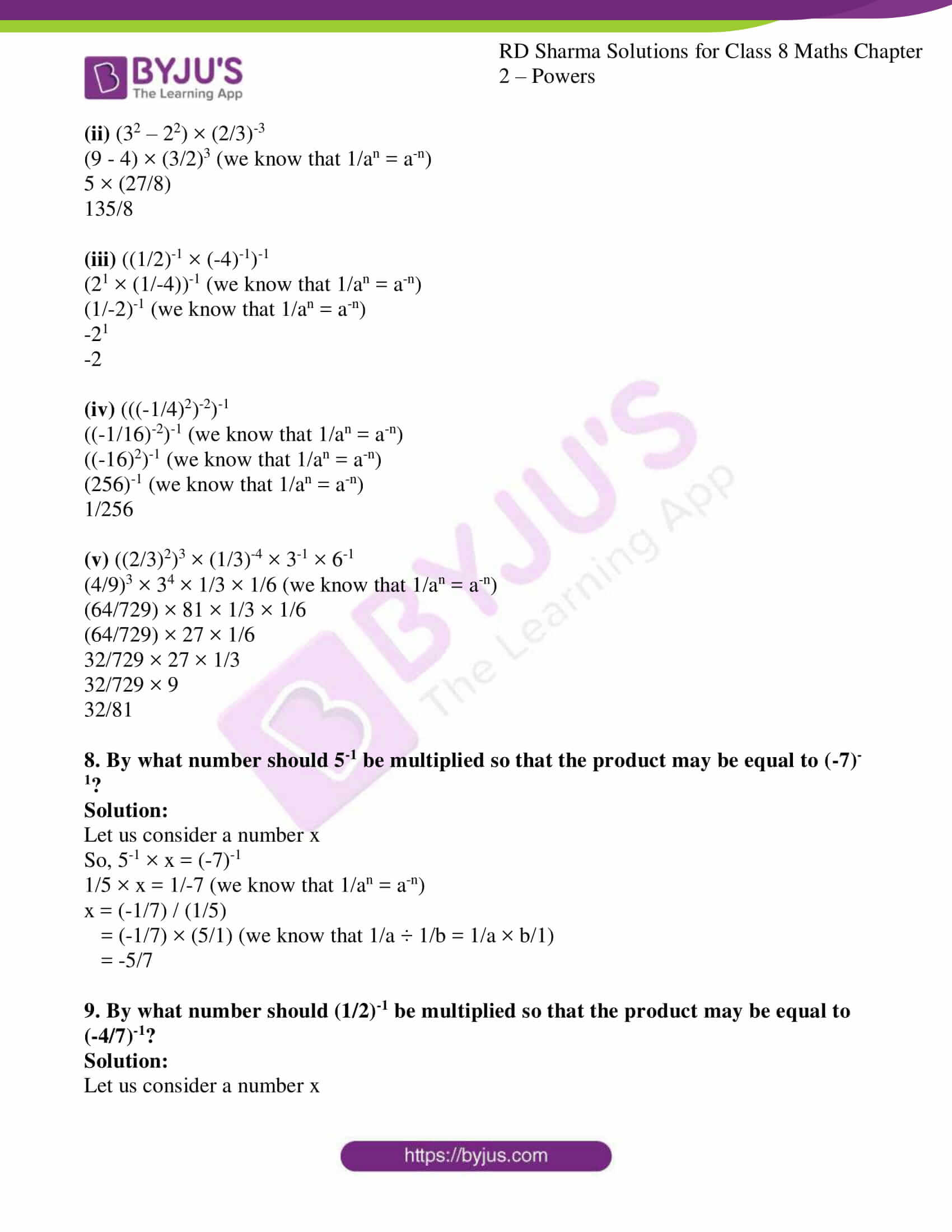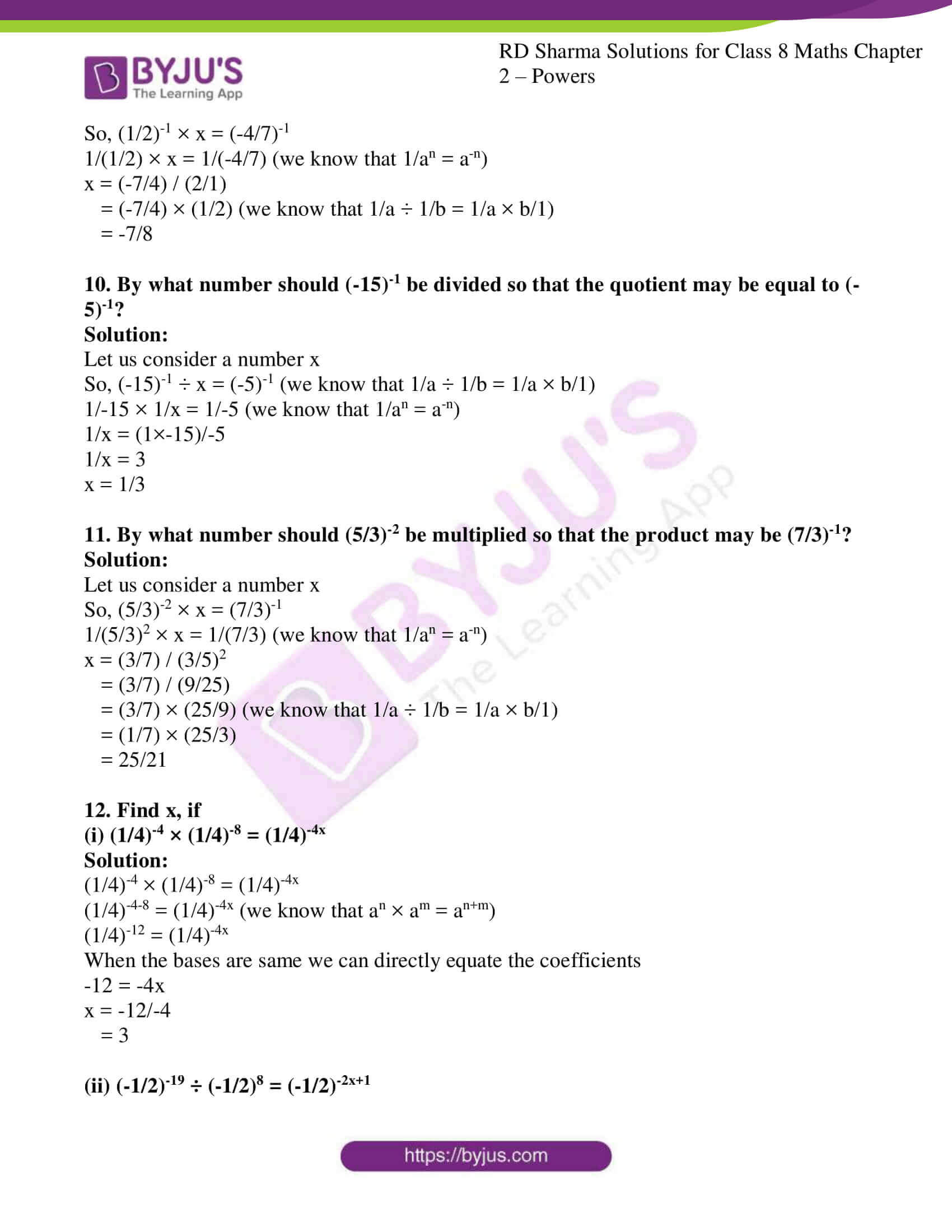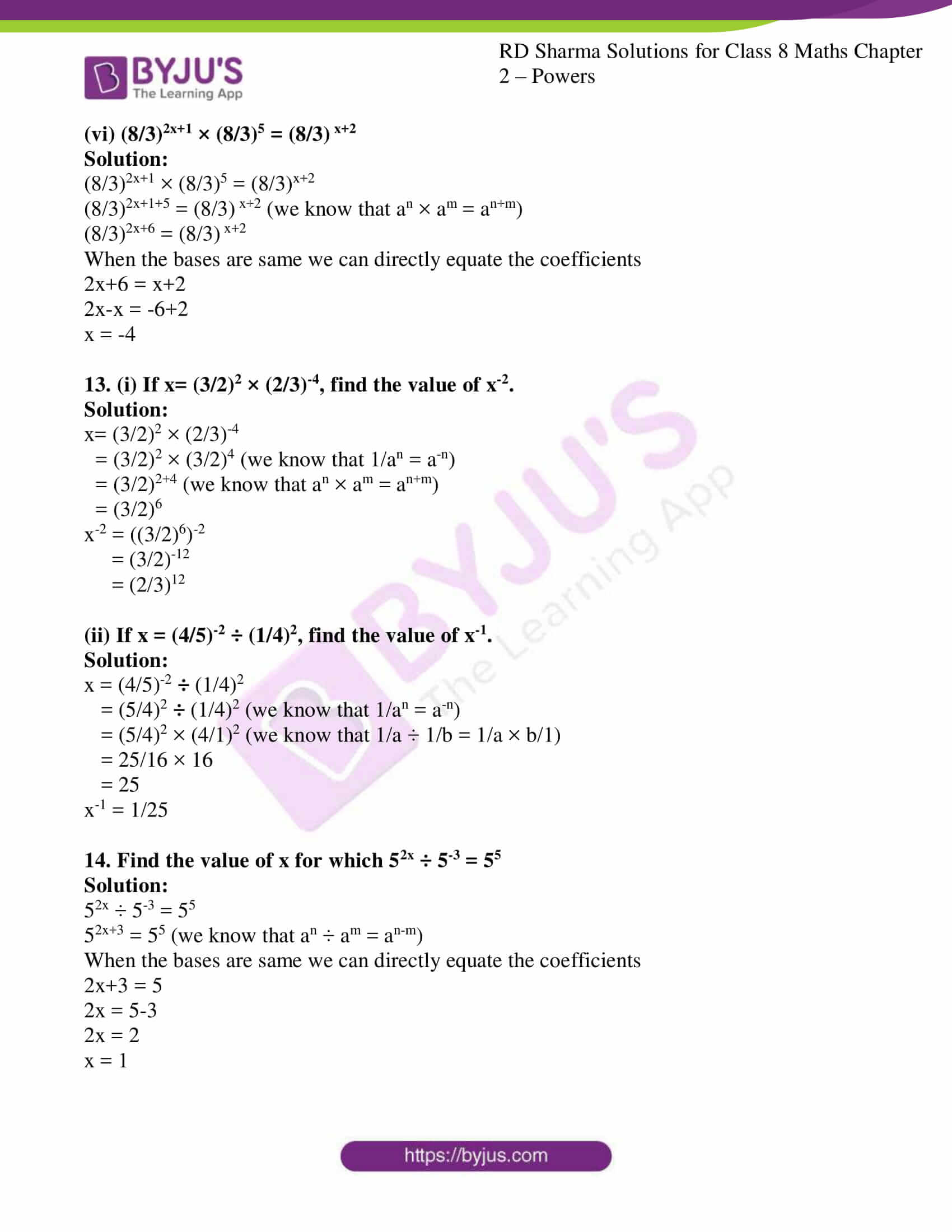### Access Answers to RD Sharma Solutions for Class 8 Maths Exercise 2.2 Chapter 2 Powers

1. Write each of the following in exponential form:

(i) (3/2)-1 × (3/2)-1 × (3/2)-1 × (3/2)-1

(ii) (2/5)-2 × (2/5)-2 × (2/5)-2

Solution:

(i) (3/2)-1 × (3/2)-1 × (3/2)-1 × (3/2)-1

(3/2)-4 (we know that a-n = 1/an, an = a×a…n times)

(ii) (2/5)-2 × (2/5)-2 × (2/5)-2

(2/5)-6 (we know that a-n = 1/an, an = a×a…n times)

2. Evaluate:

(i) 5-2

(ii) (-3)-2

(iii) (1/3)-4

(iv) (-1/2)-1

Solution:

(i) 5-2

1/52 = 1/25 (we know that a-n = 1/an)

(ii) (-3)-2

(1/-3)2 = 1/9 (we know that a-n = 1/an)

(iii) (1/3)-4

34 = 81 (we know that 1/a-n = an)

(iv) (-1/2)-1

-21 = -2 (we know that 1/a-n = an)

3. Express each of the following as a rational number in the form p/q:

(i) 6-1

(ii) (-7)-1

(iii) (1/4)-1

(iv) (-4)-1 × (-3/2)-1

(v) (3/5)-1 × (5/2)-1

Solution:

(i) 6-1

1/61 = 1/6 (we know that a-n = 1/an)

(ii) (-7)-1

1/-71 = -1/7 (we know that a-n = 1/an)

(iii) (1/4)-1

41 = 4 (we know that 1/a-n = an)

(iv) (-4)-1 × (-3/2)-1

1/-41 × (2/-3)1 (we know that a-n = 1/an, 1/a-n = an)

1/-2 × -1/3

1/6

(v) (3/5)-1 × (5/2)-1

(5/3)1 × (2/5)1

5/3 × 2/5

2/3

4. Simplify:

(i) (4-1 × 3-1)2

(ii) (5-1 ÷ 6-1)3

(iii) (2-1 + 3-1)-1

(iv) (3-1 × 4-1)-1 × 5-1

(v) (4-1 – 5-1) ÷ 3-1

Solution:

(i) (4-1 × 3-1)2

(1/4 × 1/3)2 (we know that a-n = 1/an)

(1/12)2

1/144

(ii) (5-1 ÷ 6-1)3

(1/5 ÷ 1/6)3 (we know that a-n = 1/an)

(1/5 × 6)3 (we know that 1/a ÷ 1/b = 1/a × b/1)

(6/5)3

216/125

(iii) (2-1 + 3-1)-1

(1/2 + 1/3)-1 (we know that a-n = 1/an)

LCM of 2 and 3 is 6

((3+2)/6)-1

(5/6)-1 (we know that 1/a-n = an)

6/5

(iv) (3-1 × 4-1)-1 × 5-1

(1/3 × 1/4)-1 × 1/5 (we know that a-n = 1/an)

(1/12)-1 × 1/5 (we know that 1/a-n = an)

12 × 1/5

12/5

(v) (4-1 – 5-1) ÷ 3-1

(1/4 – 1/5) ÷ 1/3 (we know that a-n = 1/an)

LCM of 4 and 5 is 20

(5-4)/20 × 3/1 (we know that 1/a ÷ 1/b = 1/a × b/1)

1/20 × 3

3/20

5. Express each of the following rational numbers with a negative exponent:

(i) (1/4)3

(ii) 35

(iii) (3/5)4

(iv) ((3/2)4)-3

(v) ((7/3)4)-3

Solution:

(i) (1/4)3

(4)-3 (we know that 1/an = a-n)

(ii) 35

(1/3)-5 (we know that 1/an = a-n)

(iii) (3/5)4

(5/3)-4 (we know that (a/b)-n = (b/a)n)

(iv) ((3/2)4)-3

(3/2)-12 (we know that (an)m = anm)

(v) ((7/3)4)-3

(7/3)-12 (we know that (an)m = anm)

6. Express each of the following rational numbers with a positive exponent:

(i) (3/4)-2

(ii) (5/4)-3

(iii) 43 × 4-9

(iv) ((4/3)-3)-4

(v) ((3/2)4)-2

Solution:

(i) (3/4)-2

(4/3)2 (we know that (a/b)-n = (b/a)n)

(ii) (5/4)-3

(4/5)3 (we know that (a/b)-n = (b/a)n)

(iii) 43 × 4-9

(4)3-9 (we know that an × am = an+m)

4-6

(1/4)6 (we know that 1/an = a-n)

(iv) ((4/3)-3)-4

(4/3)12 (we know that (an)m = anm)

(v) ((3/2)4)-2

(3/2)-8 (we know that (an)m = anm)

(2/3)8 (we know that 1/an = a-n)

7. Simplify:

(i) ((1/3)-3 – (1/2)-3) ÷ (1/4)-3

(ii) (32 – 22) × (2/3)-3

(iii) ((1/2)-1 × (-4)-1)-1

(iv) (((-1/4)2)-2)-1

(v) ((2/3)2)3 × (1/3)-4 × 3-1 × 6-1

Solution:

(i) ((1/3)-3 – (1/2)-3) ÷ (1/4)-3

(33 – 23) ÷ 43 (we know that 1/an = a-n)

(27-8) ÷ 64

19 ÷ 64

19 × 1/64 (we know that 1/a ÷ 1/b = 1/a × b/1)

19/64

(ii) (32 – 22) × (2/3)-3

(9 – 4) × (3/2)3 (we know that 1/an = a-n)

5 × (27/8)

135/8

(iii) ((1/2)-1 × (-4)-1)-1

(21 × (1/-4))-1 (we know that 1/an = a-n)

(1/-2)-1 (we know that 1/an = a-n)

-21

-2

(iv) (((-1/4)2)-2)-1

((-1/16)-2)-1 (we know that 1/an = a-n)

((-16)2)-1 (we know that 1/an = a-n)

(256)-1 (we know that 1/an = a-n)

1/256

(v) ((2/3)2)3 × (1/3)-4 × 3-1 × 6-1

(4/9)3 × 34 × 1/3 × 1/6 (we know that 1/an = a-n)

(64/729) × 81 × 1/3 × 1/6

(64/729) × 27 × 1/6

32/729 × 27 × 1/3

32/729 × 9

32/81

8. By what number should 5-1 be multiplied so that the product may be equal to (-7)-1?

Solution:

Let us consider a number x

So, 5-1 × x = (-7)-1

1/5 × x = 1/-7 (we know that 1/an = a-n)

x = (-1/7) / (1/5)

= (-1/7) × (5/1) (we know that 1/a ÷ 1/b = 1/a × b/1)

= -5/7

9. By what number should (1/2)-1 be multiplied so that the product may be equal to (-4/7)-1?

Solution:

Let us consider a number x

So, (1/2)-1 × x = (-4/7)-1

1/(1/2) × x = 1/(-4/7) (we know that 1/an = a-n)

x = (-7/4) / (2/1)

= (-7/4) × (1/2) (we know that 1/a ÷ 1/b = 1/a × b/1)

= -7/8

10. By what number should (-15)-1 be divided so that the quotient may be equal to (-5)-1?

Solution:

Let us consider a number x

So, (-15)-1 ÷ x = (-5)-1 (we know that 1/a ÷ 1/b = 1/a × b/1)

1/-15 × 1/x = 1/-5 (we know that 1/an = a-n)

1/x = (1×-15)/-5

1/x = 3

x = 1/3

11. By what number should (5/3)-2 be multiplied so that the product may be (7/3)-1?

Solution:

Let us consider a number x

So, (5/3)-2 × x = (7/3)-1

1/(5/3)2 × x = 1/(7/3) (we know that 1/an = a-n)

x = (3/7) / (3/5)2

= (3/7) / (9/25)

= (3/7) × (25/9) (we know that 1/a ÷ 1/b = 1/a × b/1)

= (1/7) × (25/3)

= 25/21

12. Find x, if

(i) (1/4)-4 × (1/4)-8 = (1/4)-4x

Solution:

(1/4)-4 × (1/4)-8 = (1/4)-4x

(1/4)-4-8 = (1/4)-4x (we know that an × am = an+m)

(1/4)-12 = (1/4)-4x

When the bases are same we can directly equate the coefficients

-12 = -4x

x = -12/-4

= 3

(ii) (-1/2)-19 ÷ (-1/2)8 = (-1/2)-2x+1

Solution:

(-1/2)-19 ÷ (-1/2)8 = (-1/2)-2x+1

(1/2)-19-8 = (1/2)-2x+1 (we know that an ÷ am = an-m)

(1/2)-27 = (1/2)-2x+1

When the bases are same we can directly equate the coefficients

-27 = -2x+1

-2x = -27-1

x = -28/-2

= 14

(iii) (3/2)-3 × (3/2)5 = (3/2)2x+1

Solution:

(3/2)-3 × (3/2)5 = (3/2)2x+1

(3/2)-3+5 = (3/2)2x+1 (we know that an × am = an+m)

(3/2)2 = (3/2)2x+1

When the bases are same we can directly equate the coefficients

2 = 2x+1

2x = 2-1

x = 1/2

(iv) (2/5)-3 × (2/5)15 = (2/5)2+3x

Solution:

(2/5)-3 × (2/5)15 = (2/5)2+3x

(2/5)-3+15 = (2/5)2+3x (we know that an × am = an+m)

(2/5)12 = (2/5)2+3x

When the bases are same we can directly equate the coefficients

12 = 2+3x

3x = 12-2

x = 10/3

(v) (5/4)-x ÷ (5/4)-4 = (5/4)5

Solution:

(5/4)-x ÷ (5/4)-4 = (5/4)5

(5/4)-x+4 = (5/4)5 (we know that an ÷ am = an-m)

When the bases are same we can directly equate the coefficients

-x+4 = 5

-x = 5-4

-x = 1

x = -1

(vi) (8/3)2x+1 × (8/3)5 = (8/3) x+2

Solution:

(8/3)2x+1 × (8/3)5 = (8/3)x+2

(8/3)2x+1+5 = (8/3) x+2 (we know that an × am = an+m)

(8/3)2x+6 = (8/3) x+2

When the bases are same we can directly equate the coefficients

2x+6 = x+2

2x-x = -6+2

x = -4

13. (i) If x= (3/2)2 × (2/3)-4, find the value of x-2.

Solution:

x= (3/2)2 × (2/3)-4

= (3/2)2 × (3/2)4 (we know that 1/an = a-n)

= (3/2)2+4 (we know that an × am = an+m)

= (3/2)6

x-2 = ((3/2)6)-2

= (3/2)-12

= (2/3)12

(ii) If x = (4/5)-2 ÷ (1/4)2, find the value of x-1.

Solution:

x = (4/5)-2 ÷ (1/4)2

= (5/4)2 ÷ (1/4)2 (we know that 1/an = a-n)

= (5/4)2 × (4/1)2 (we know that 1/a ÷ 1/b = 1/a × b/1)

= 25/16 × 16

= 25

x-1 = 1/25

14. Find the value of x for which 52x ÷ 5-3 = 55

Solution:

52x ÷ 5-3 = 55

52x+3 = 55 (we know that an ÷ am = an-m)

When the bases are same we can directly equate the coefficients

2x+3 = 5

2x = 5-3

2x = 2

x = 1

## RD Sharma Solutions for Class 8 Maths Exercise 2.2 Chapter 2 Powers

Class 8 Maths Chapter 2 Powers Exercise 2.2 is based on the problems which include all the six laws of integral exponents and use of decimal number system in the laws. To facilitate easy learning and understanding of concepts, download free RD Sharma Solutions Chapter 2 in PDF format, which provides answers to all the questions. Try solving these solutions which help students attain good marks in their academics.